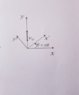# Measuring position and velocity from a non inertial reference frame

Like Tony Stark
Homework Statement:
A particle moves with constant velocity ##v_0## in the ##y## direction with respect to an inertial system ##A(x;y;z)## as depicted in the picture. There is another system ##B(x′;y′;z′)##, which is not inertial and rotates with constant angular velocity ω.
Determine
I) ##r(t)## from the perspective of ##B## and ##A##
II) the velocity measured from ##B##, i.e., the velocity relative to ##B##
III) the acceleration measured from ##B##, i.e., the acceleration relative to ##B##
Relevant Equations:
Relative motion equations for non inertial reference frame
I) For ##A##, the positition is ##\vec r=(0;V_0 . t;0)##.
For ##B##, we have ##\vec r_A=\vec r_B + \vec r_{A/B}##, but ##\vec r_{A/B}## is equal to zero because they have the same origin, so the position measured from ##A## is equal to the position measured from ##B##

II) For ##A##, velocity is equal to ##V_0##.
For ##B## we know that ##\vec V_A = \vec V_B +\vec \omega \times \vec V_{rel}##. ##\vec V_A## is equal to ##(0;V_0;0)##; ##\vec V_B=0##, since the origin doesn't move; ##\vec \omega## is equal to ##(0;0;\theta /t)##, ##\vec r## is the vector calculated previously and then I solve for ##\vec V_{rel}##.

III) For acceleration we just have to replace the values in the formula and solve for ##\vec a_{rel}##

Is this ok?

#### Attachments

•20190915_114157.jpg
15.1 KB · Views: 96

Homework Helper
Gold Member
2022 Award
Homework Statement: A particle moves with constant velocity ##v_0## in the ##y## direction with respect to an inertial system ##A(x;y;z)## as depicted in the picture. There is another system ##B(x′;y′;z′)##, which is not inertial and rotates with constant angular velocity ω.
Determine
I) ##r(t)## from the perspective of ##B## and ##A##
II) the velocity measured from ##B##, i.e., the velocity relative to ##B##
III) the acceleration measured from ##B##, i.e., the acceleration relative to ##B##
Homework Equations: Relative motion equations for non inertial reference frame

I) For ##A##, the positition is ##\vec r=(0;V_0 . t;0)##.
For ##B##, we have ##\vec r_A=\vec r_B + \vec r_{A/B}##, but ##\vec r_{A/B}## is equal to zero because they have the same origin, so the position measured from ##A## is equal to the position measured from ##B##

How can that be? In any case, you have to calculate ##r_B(t)##. Can you do that?

Hint: the axes of frames A and B are rotating wrt each other.

Like Tony Stark
How can that be? In any case, you have to calculate ##r_B(t)##. Can you do that?

Hint: the axes of frames A and B are rotating wrt each other.

I can't see why it's incorrect. I mean, I also think that the positions must be different since they're measured from different systems, but if I go to the equations I find out that ##\vec r_{A/B}## is the vector that goes from the origin of the fixed system to the origin of the rotating system, and in the picture I see that both systems share the same origin, and it's always like that since ##B## rotates but its origin doesn't move.

#### Attachments

Homework Helper
Gold Member
2022 Award
I can't see why it's incorrect. I mean, I also think that the positions must be different since they're measured from different systems, but if I go to the equations I find out that ##\vec r_{A/B}## is the vector that goes from the origin of the fixed system to the origin of the rotating system, and in the picture I see that both systems share the same origin, and it's always like that since ##B## rotates but its origin doesn't move.
If an object is at rest in frame A, is it at rest in frame B?

Like Tony Stark
If an object is at rest in frame A, is it at rest in frame B?
No, because of the angular velocity. I can notice that, from frame ##B##, the particle moves so the position vector is changing. I understand that. But my doubt is about the equation: what's wrong with saying that the vector from one system to another is zero?

Homework Helper
Gold Member
2022 Award
No, because of the angular velocity. I can notice that, from frame ##B##, the particle moves so the position vector is changing. I understand that. But my doubt is about the equation: what's wrong with saying that the vector from one system to another is zero?

That's only valid for translations. Rotations entail a different transformation between coordinates.

Like Tony Stark
That's only valid for translations. Rotations entail a different transformation between coordinates.
Ok. But apart from that, is the solution for velocity and acceleration ok? (Obviously, I have to change the values for position, but without considering that mistake)

Homework Helper
Gold Member
2022 Award
Ok. But apart from that, is the solution for velocity and acceleration ok? (Obviously, I have to change the values for position, but without considering that mistake)
I don't see a clear solution for those, but I'm not convinced that you understand the rotation transformation from what you've written.

Like Tony Stark
I don't see a clear solution for those, but I'm not convinced that you understand the rotation transformation from what you've written.
I've changed basis. I wrote the position of the particle in terms of the basis vectors of ##B##. Now I have to replace this new values in the ##\vec r## part of the equation of velocity and acceleration. Right?